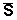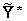# 交流電路供電單擊或點擊下面的示例電路以調用TINACloud並選擇交互式DC模式以在線分析它們。

1. 瞬時功率： P（T） 是權力的時間函數， p（t）= u（t）* i（t）。 它是電壓和電流的時間函數的乘積。 瞬時功率的定義適用於任何波形的信號。 的單位 瞬時功率 是VA。

2. 複數功率： S3. 真實 or 平均功率: P 可以用兩種方式定義：作為複數冪的實部或作為 瞬時功率。 第二個定義比較籠統，因為我們可以用它來定義 瞬時功率 適用於任何信號波形，而不僅僅是正弦波。 在以下表達式中明確給出4. 無功功率： Q 是複雜力量的虛構部分。 單位為 伏安無功 （VAR）。 無功功率 積極感應的 電路電容電路。 該功率僅限於正弦激勵。 無功功率不做任何有用的工作或發熱量， 是電路的電抗組件（電感器，電容器）返回電源的功率

5. 視在功率： S 是電壓和電流均方根值的乘積，S = U * I。 視在功率的單位是VA。 的 視在功率 是絕對值 複雜的力量，因此僅定義為正弦激勵。

###### 強大能力 因子 （COS φ)TINA的功率測量儀器還可以測量功率因數。= 3.9 ej38.7BмmA

PR= I2* R =（3.052+ 2.442）* 2 / 2 = 15.2 mW

QC = -I2/wC = -15.2 / 1.256 = -12.1mVARpV（t）= - vS（t）* i（t）= -10 cosωt* 3.9 cos（ωt + 38.7 м）= -39cosωt *（cosωt cos 38.7 м-sinωt sin 38.7 м ）= -30.45 cosωt + 24.4 sinωtVAS = V.S* I = 10 * 3.9 / 2 = 19.5 VA

{TINA口譯員的解決方案}
OM：= 2 * PI * 1000;

I：= V /（R + 1 /（j * OM * C））;
IAQ：= SQR（ABS（I））;
PR：= IAQ * R / 2;
PR = [15.3068m]
QC：= IAQ /（OM * C * 2）;
QC = [12.1808m]
IC：=的Re（I）-j * IM（I）;
SV：= - V * IC / 2;
SV = [ - + 15.3068m * 12.1808m j]的
#Python解決方案

#讓我們簡化複雜的打印
#numbers 提高透明度：
cp= lambda Z : “{:.4f}”.format(Z)
om=2000*c.pi
V=10
I=V/(R+1/1j/om/C)
laq=abs(I)**2
PR=laq*R/2

QC=laq/om/C/2

Ic=I.共軛()
Sv=-V*Ic/2

P QS R * I2 X *我2 ½Z½* I2 Z*I2 g ^ * V2 -B * V2 ½Y½* V2V2

#### 例如2

iS（t）=（100 * cosωt）mA w = 1 krad / s

Yeq= 1 / R + jωC + 1 / jωL = 1/5 + j250 * 10-6103 + 1 /（j * 20 * 10-3103）= 0.2 + j0.2 S.

½V ½= ½Z ½* I = I / ½Y ½= 0.1 / ê（0.2 + j0.2） ê= 0.3535 V.

P = V.2* G = 0.125 * 0.2 / 2 = 0.0125 W.

Q = -V2* B = – 0.125 * 0.2 / 2 = – 0.0125 var= V.2*= 0.125 *（0.2-j0.2）/ 2 =（12.5 – j 12.5）mVA

S = V.2* Y = 0.125 * ê0.2 + j0.2 ê/ 2 = 0.01768 VA

cosφ= P / S = 0.707{TINA口譯員的解決方案}
OM：= 1000;

V = [250m-250m * j]的
S：= V *是/ 2;
S = [12.5m-12.5m * j]的

P = [12.5m]
Q = [ - 12.5m]
ABS（S）= [17.6777m]
#Python解決方案
#讓我們簡化複雜的打印
#numbers 提高透明度：
cp= lambda Z : “{:.4f}”.format(Z)

V=Is*(1/(1/R+1j*om*C+1/1j/om/L))

S=V*Is/2
P=S.實數
Q=S.imag

print(“abs(S)=”,cp(abs(S))){TINA口譯員的解決方案！}
VS：= 100;
OM：= 1E8 * 2 * PI;
Ie:=Vs/(R2+1/j/om/C2+replus(replus(R1,j*om*L),1/j/om/C1));
Ze:=(R2+1/j/om/C2+replus(replus(R1,j*om*L),1/j/om/C1));

P = [14.6104]
Q = [ - 58.7055]
#Python解決方案

#讓我們簡化複雜的打印
#numbers 提高透明度：
cp= lambda Z : “{:.4f}”.format(Z)
#使用 lambda 定義 replus：
Replus= 拉姆達 R1, R2 : R1*R2/(R1+R2)
VS=100
om=200000000*c.pi
Ie=Vs/(R2+1/1j/om/C2+Replus(Replus(R1,1j*om*L),1/1j/om/C1))
Ze=R2+1/1j/om/C2+Replus(Replus(R1,1j*om*L),1/1j/om/C1)
p=abs(Ie)**2*Ze.real/2

X欢迎 DesignSoft
如果需要任何幫助找到合適的產品或需要支持，可以進行聊天。